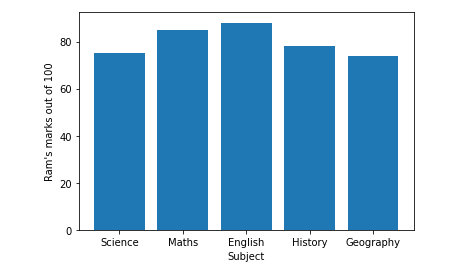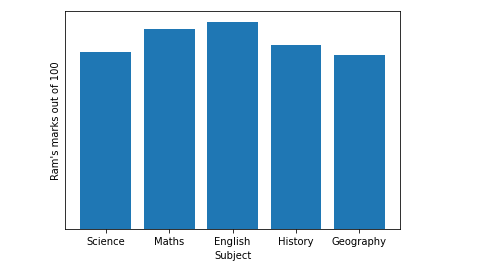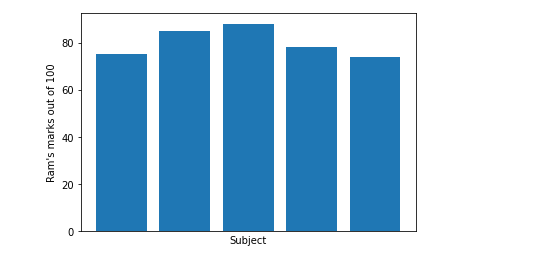# Hide Axis, Borders and White Spaces in Matplotlib

When we draw plots using Matplotlib, the ticks and labels along x-axis & y-axis are drawn too.  For drawing creative graphs, many times we hide x-axis & y-axis.

## How to hide axis in matplotlib figure?

The matplotlib.pyplot.axis(‘off’) command us used to hide the axis(both x-axis & y-axis) in the matplotlib figure.

Example:

Let us consider the following figure in which we have to hide the axis.

## Python3

 `# code ` `import` `numpy as np ` `import` `matplotlib.pyplot as plt ` ` `  `# Marks of RAM in different subjects out of 100. ` `x ``=` `[``'Science'``, ``'Maths'``, ``'English'``, ``'History'``, ``'Geography'``] ` `y ``=` `[``75``, ``85``, ``88``, ``78``, ``74``] ` ` `  `plt.bar(x, y) ` `plt.xlabel(``"Subject"``) ` `plt.ylabel(``"Ram's marks out of 100"``) ` `plt.show() `

Output:Example:

Hiding the axis in the above figure.

## Python3

 `# code ` `import` `numpy as np ` `import` `matplotlib.pyplot as plt ` ` `  `# Marks of RAM in different subjects out of 100. ` `x ``=` `[``'Science'``, ``'Maths'``, ``'English'``, ``'History'``, ``'Geography'``] ` `y ``=` `[``75``, ``85``, ``88``, ``78``, ``74``] ` ` `  `plt.xlabel(``"Subject"``) ` `plt.ylabel(``"Ram's marks out of 100"``) ` `plt.bar(x, y) ` `plt.axis(``'off'``)  ``# command for hiding the axis. ` ` `  `plt.show() `

Output:If we just want to turn either the X-axis or Y-axis off, we can use  plt.xticks( ) or plt.yticks( )  method respectively.

Example:

Hiding Y-axis

## Python3

 `# Hiding Y-axis label ` `import` `numpy as np ` `import` `matplotlib.pyplot as plt ` ` `  `# Marks of RAM in different subjects out of 100. ` `x ``=` `[``'Science'``, ``'Maths'``, ``'English'``, ``'History'``, ``'Geography'``] ` `y ``=` `[``75``, ``85``, ``88``, ``78``, ``74``] ` ` `  `plt.bar(x, y) ` `plt.xlabel(``"Subject"``) ` `plt.ylabel(``"Ram's marks out of 100"``) ` `plt.yticks([])  ``# Command for hiding y-axis ` ` `  `plt.show() `

Output:Example:

Hiding X-axis

## Python3

 `# Hiding X-axis ` `import` `numpy as np ` `import` `matplotlib.pyplot as plt ` ` `  `# Marks of RAM in different subjects out of 100. ` `x ``=` `[``'Science'``, ``'Maths'``, ``'English'``, ``'History'``, ``'Geography'``] ` `y ``=` `[``75``, ``85``, ``88``, ``78``, ``74``] ` ` `  `plt.bar(x, y) ` `plt.xlabel(``"Subject"``) ` `plt.ylabel(``"Ram's marks out of 100"``) ` `plt.xticks([])  ``# Command for hiding x-axis ` ` `  `plt.show() `

Output:## Hiding the Whitespaces and Borders in the Matplotlib figure

When we use plt.axis(‘off’) command it hides the axis, but we get whitespaces around the image’s border while saving it. To remove/hide whitespace around the border, we can set bbox_inches=’tight’ in the savefig() method.

Similarly, to remove the white border around the image while we set pad_inches = 0 in the savefig() method.

Example:

## Python3

 `# code ` `import` `numpy as np ` `import` `matplotlib.pyplot as plt ` ` `  `# Marks of RAM in different subjects out of 100. ` `x ``=` `[``'Science'``, ``'Maths'``, ``'English'``, ``'History'``, ``'Geography'``] ` `y ``=` `[``75``, ``85``, ``88``, ``78``, ``74``] ` ` `  `fig ``=` `plt.bar(x, y) ` `plt.xlabel(``"Subject"``) ` `plt.ylabel(``"Ram's marks out of 100"``) ` `plt.axis(``'off'``) ` `# Command used for hiding whitespaces and border. ` `plt.savefig(``'image.png'``, bbox_inches``=``'tight'``, pad_inches``=``0``) ` ` `  `plt.show() `

Output:Note: If you have noticed that when we use plt.axis(‘off’) it automatically hides the Axis, Whitespaces and Borders.

Whether you're preparing for your first job interview or aiming to upskill in this ever-evolving tech landscape, GeeksforGeeks Courses are your key to success. We provide top-quality content at affordable prices, all geared towards accelerating your growth in a time-bound manner. Join the millions we've already empowered, and we're here to do the same for you. Don't miss out - check it out now!

Previous
Next